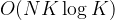# Teaching Kids Programming – Two Algorithms to Group Anagrams

Teaching Kids Programming: Videos on Data Structures and Algorithms

Given an array of strings strs, group the anagrams together. You can return the answer in any order. An Anagram is a word or phrase formed by rearranging the letters of a different word or phrase, typically using all the original letters exactly once.

Example 1:
Input: strs = [“eat”,”tea”,”tan”,”ate”,”nat”,”bat”]
Output: [[“bat”],[“nat”,”tan”],[“ate”,”eat”,”tea”]]

Example 2:
Input: strs = [“”]
Output: [[“”]]

Example 3:
Input: strs = [“a”]
Output: [[“a”]]

Constraints:
1 <= strs.length <= 10^4
0 <= strs[i].length <= 100
strs[i] consists of lowercase English letters.

### Group Anagrams via Sorting and Hash Table aka Dictionary

After sorting, the same group anagram strings will give a same string. We then can use this as a unique key to identify the anagram group. There are N words, and if the maximum word length is K, we need O(KLogK) to sort the words, the overall time complexity is$tex_652d85de047af8a668617046c2c1c9e1 Teaching Kids Programming - Two Algorithms to Group Anagrams algorithms python teaching kids programming youtube video$. The space complexity is O(NK) as we use a hash table to store the anagram groups.

 ```1 2 3 4 5 6 ``` ```class Solution:     def groupAnagrams(self, strs: List[str]) -> List[List[str]]:         data = defaultdict(list)         for s in strs:             data[tuple(sorted(s))].append(s)         return data.values()```
```class Solution:
def groupAnagrams(self, strs: List[str]) -> List[List[str]]:
data = defaultdict(list)
for s in strs:
data[tuple(sorted(s))].append(s)
return data.values()```

We can convert the list (sorted) to tuple and use it as a key. The default dictionary has default value type list.

### Group Anagrams via Counting and Hash Table aka Dictionary

Recall that we can use Counter to compare equality of two anagram strings – However, we cannot convert Counter to tuple as it gives incorrect equality test. Given the words are lowercase, we can count and store the frequencies in an array/list.

 ```1 2 3 4 5 6 7 8 9 ``` ```class Solution:     def groupAnagrams(self, strs: List[str]) -> List[List[str]]:         data = defaultdict(list)         for s in strs:             d =  * 26             for w in s:                 d[ord(w) - 97] += 1             data[tuple(d)].append(s)         return data.values()```
```class Solution:
def groupAnagrams(self, strs: List[str]) -> List[List[str]]:
data = defaultdict(list)
for s in strs:
d =  * 26
for w in s:
d[ord(w) - 97] += 1
data[tuple(d)].append(s)
return data.values()```

The time complexity is O(NK). so as the space complexity.

GD Star Rating